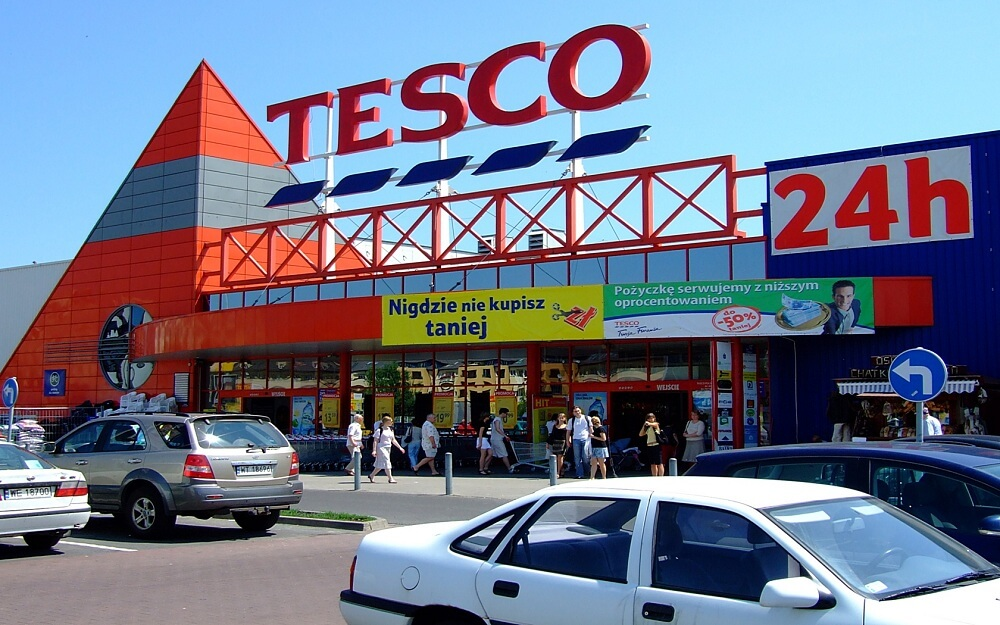# What is Price elasticity of demand?13/10/2016Ángela de la Vieja

We are sure that you will have heard on many occasions this popular term in the pricing world, but maybe you don´t know exactly what it means. In a very short form and without going into mathematical explanations we are going to explain the term with some examples.

Price elasticity of demand (PED) refers to the elasticity or evolution of the demand of a certain product or service when it´s price varies. It is represented by a curve on a graph where axis (Y) indicates the price and axis (X) the demand:Let’s put this into an example to understand it better. Imagine a very popular consumer product that has a punctual discount applied, the elasticity implies that a reduction in price means a much larger increase in demand and therefore we can say that this product has a high elasticity.  However, imagine applying the exact same discount to a product that is a lot less known; the demand hardly increases and therefore we can say that it is inelastic.

Put in another way, with the first example we would gain profit because the increase in demand would compensate the margin loss, whereas with the second example, the discount would result in being negative as we would sell slightly more but not enough to compensate the margin loss.

Measuring this concept in our e commerce could be very beneficial to us, as to understand how and when we should apply discounts to our products.

Find out how Minderest can take your business to the next level.

Contact our pricing experts to see the platform in action.